# The Triangular Cupola

The triangular cupola is one of the Johnson solids. It is bounded by 4 equilateral triangles, 3 squares, and a regular hexagon, for a total of 8 faces, 15 edges, and 9 vertices. It is the third polyhedron in Norman Johnson's list, and thus bears the label J3.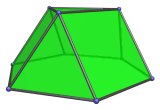The triangular cupola can be constructed by bisecting the cuboctahedron between two opposite triangular faces. Conversely, two triangular cupolae can be glued together in gyro orientation to produce a cuboctahedron: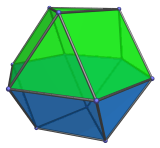If two triangular cupolae are glued together in ortho orientation instead, the result is a triangular orthobicupola (J27), another Johnson solid: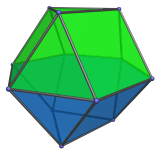The triangular cupola can also be lengthened by attaching a hexagonal prism, forming the elongated triangular cupola (J18). Adding a second cupola in ortho orientation produces the elongated triangular orthobicupola (J35); adding a second cupola in gyro orientation produces the elongated triangular gyrobicupola (J36).

It can also be lengthened by attaching a hexagonal antiprism, producing the gyroelongated triangular cupola (J22). Adding a second cupola produces the gyroelongated triangular bicupola (J44), a chiral polyhedron.

The triangular cupola can augment the truncated tetrahedron to produce the augmented truncated tetrahedron (J65).

## Projections

In order to be able to identify the triangular cupola in various projections of 4D objects, it is useful to know how it appears from various viewpoints. The following are some of the viewpoints that are commonly encountered:

Projection Envelope Description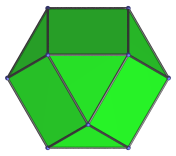Regular hexagon

Parallel projection centered on top triangular face.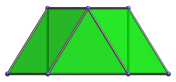Trapezium

Projection parallel to a pair of opposite edges of the hexagon.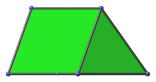Trapezium

Projection parallel to a pair of opposite vertices of the hexagon.

## Coordinates

The coordinates of the triangular cupola are:

• (±2, 0, 0)
• (±1, ±√3, 0)
• (0, 2/√3, √(8/3))
• (±1, −1/√3, √(8/3))

These coordinates describe a triangular cupola with edge length 2, resting on the XY plane with its axis of symmetry aligned to the Z axis.

Alternative coordinates can be obtained by exploiting the fact that the triangular cupola arises as a bisection of the cuboctahedron. This bisection amounts to deleting the vertices of a triangular face, so deleting the vertices of the triangle in the (−1, −1, −1) octant produces these coordinates for the triangular cupola:

 (0, ±√2, √2) (0, √2, −√2) (±√2, 0, √2) (√2, 0, −√2) (±√2, √2, 0) (√2, −√2, 0)

The triangular cupola described by these coordinates, however, is not in a “nice” orientation, as its hexagonal face is not perpendicular to the coordinate axes.

## Occurrences

The triangular cupola occurs in the following CRF polychora:

Last updated 18 Jun 2019.